faster rcnn的损失函数理解

1. 使用Smoooh L1 Loss的原因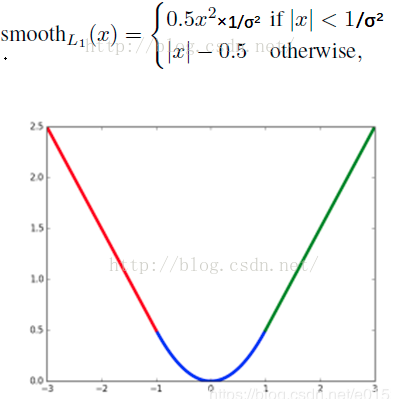2. faster rcnn的损失函数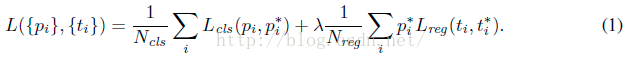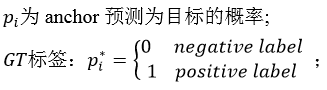2.1 分类损失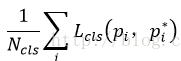其中：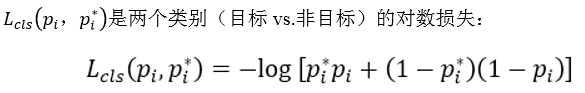2.2 bbox regression损失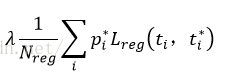•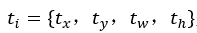是一个向量，表示该anchor预测的偏移量
•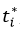是与ti维度相同的向量，表示anchor相对于gt实际的偏移量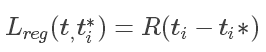R是smoothL1 函数，就是我们上面说的，不同之处是这里σ = 3，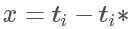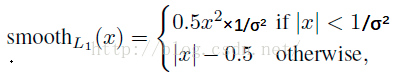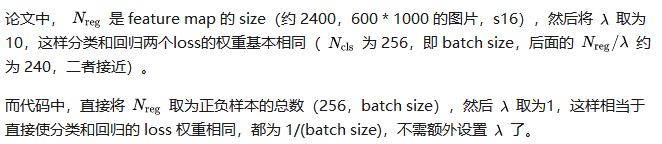2.3 tensorflow实现

    def _smooth_l1_loss(self, bbox_pred, bbox_targets, bbox_inside_weights, bbox_outside_weights, sigma=1.0, dim=):
sigma_2 = sigma ** 2
box_diff = bbox_pred - bbox_targets #ti-ti*
in_box_diff = bbox_inside_weights * box_diff  #前景才有计算损失的资格
abs_in_box_diff = tf.abs(in_box_diff) #x = |ti-ti*|
smoothL1_sign = tf.stop_gradient(tf.to_float(tf.less(abs_in_box_diff, 1. / sigma_2))) #判断smoothL1输入的大小，如果x = |ti-ti*|小于就返回1，否则返回0
#计算smoothL1损失
in_loss_box = tf.pow(in_box_diff, 2) * (sigma_2 / 2.) * smoothL1_sign + (abs_in_box_diff - (0.5 / sigma_2)) * (1. - smoothL1_sign)
out_loss_box = bbox_outside_weights * in_loss_box
loss_box = tf.reduce_mean(tf.reduce_sum(
out_loss_box,
axis=dim
))
return loss_box

一些感悟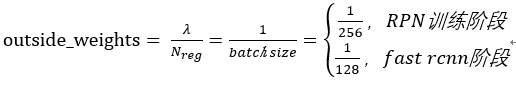# RCNN, class loss
cls_score = self._predictions["cls_score"]
label = tf.reshape(self._proposal_targets["labels"], [-1])

cross_entropy = tf.reduce_mean(
tf.nn.sparse_softmax_cross_entropy_with_logits(
logits=tf.reshape(cls_score, [-1, self._num_classes]), labels=label))

# RCNN, bbox loss
bbox_pred = self._predictions['bbox_pred'] #(128,12)
bbox_targets = self._proposal_targets['bbox_targets'] #(128,12)
bbox_inside_weights = self._proposal_targets['bbox_inside_weights']#(128,12)
bbox_outside_weights = self._proposal_targets['bbox_outside_weights']#(128,12)

loss_box = self._smooth_l1_loss(bbox_pred, bbox_targets, bbox_inside_weights, bbox_outside_weights)